# 最大熵原理

H(P)=xP(x)logP(x)$H(P)=-\sum\limits_{x}P(x)\log P(x)$

0H(P)log|X|$0\le H(P)\le\log |X|$

# 最大熵模型的定义

f(x,y)={10xy$f(x,y)= \begin{cases} 1& \text{x与y满足某一事实}\\ 0& \text{否则} \end{cases}$

EP˜(f)=x,yP˜(x,y)f(x,y)$E_{\widetilde P}(f)=\sum\limits_{x,y}\widetilde P(x,y)f(x,y)$

EP(f)=x,yP˜(x)P(y|x)f(x,y)$E_{ P}(f)=\sum\limits_{x,y} \widetilde P(x)P(y|x)f(x,y)$

Ep(f)=EP˜(f)(6.10)$E_{p}(f)=E_{\widetilde P}(f)\text{(6.10)}$

x,yP˜(x)P(y|x)f(x,y)=x,yP˜(x,y)f(x,y)(6.11)$\sum\limits_{x,y}\widetilde P(x)P(y|x)f(x,y)=\sum\limits_{x,y}\widetilde P(x,y)f(x,y)\text{(6.11)}$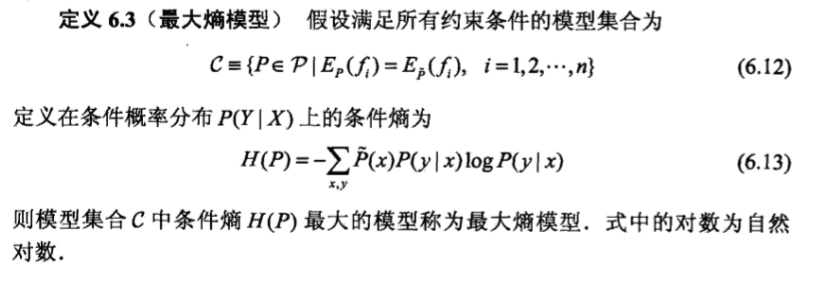# 最大熵模型的学习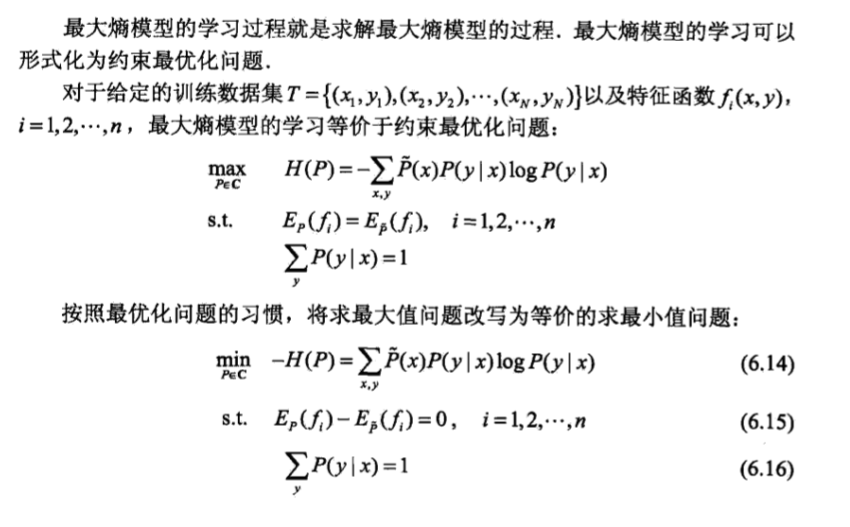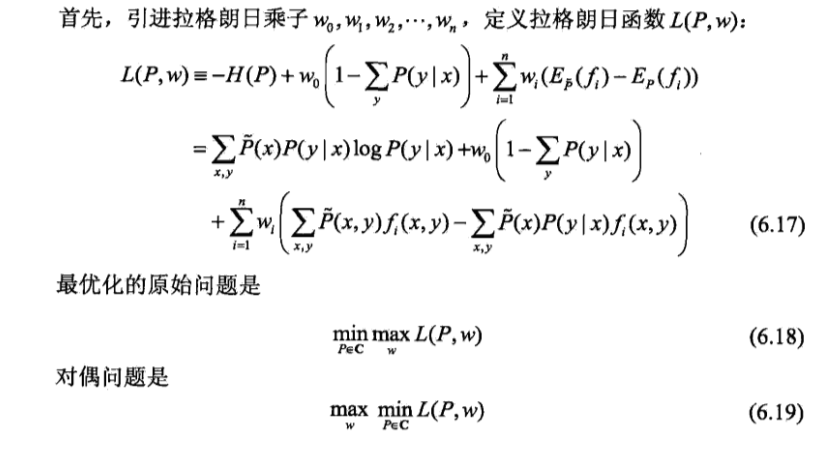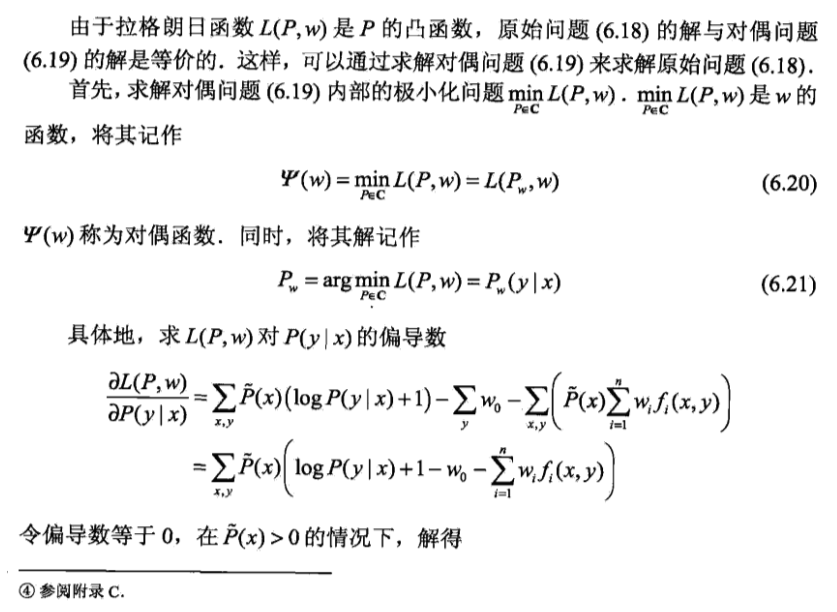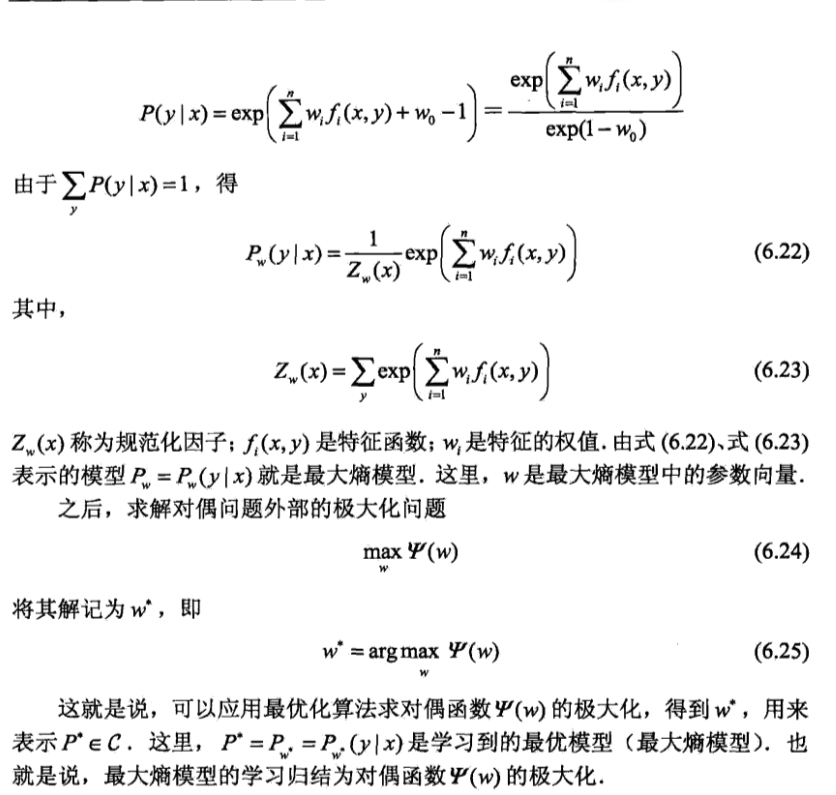# 极大似然估计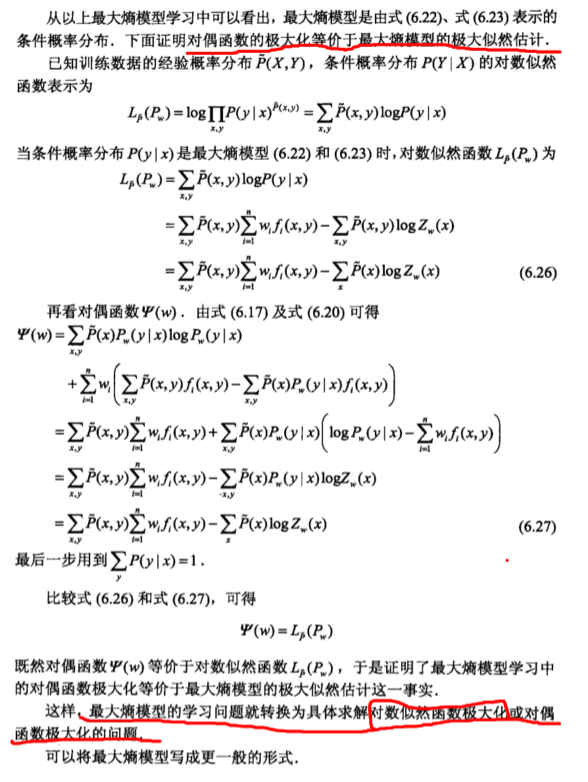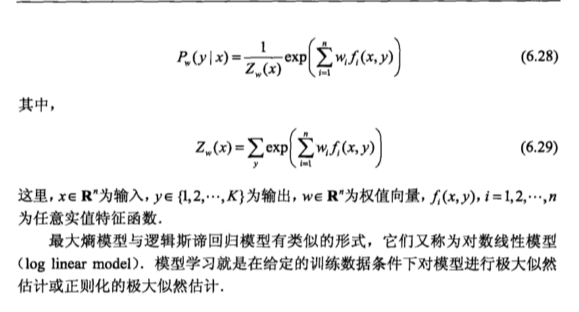【机器学习】Logistic Regression 的前世今生（理论篇）